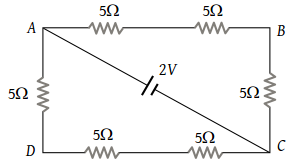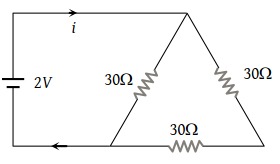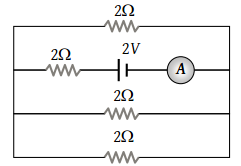A resistance R is stretched to four times its length. Its new resistance will be

(1) 4 R

(2) 64 R

(3) R/4

(4) 16 R

Concept Questions :-

Derivation of Ohm's law
High Yielding Test Series + Question Bank - NEET 2020

Difficulty Level:

What is the resistance of a carbon resistance which has bands of colours brown, black and brown

(1) 100 Ω

(2) 1000 Ω

(3) 10 Ω

(4) 1 Ω

Concept Questions :-

Colour coding of resistors
High Yielding Test Series + Question Bank - NEET 2020

Difficulty Level:

The electric resistance of a certain wire of iron is R. If its length and radius are both doubled, then :

(1) The resistance will be doubled and the specific resistance will be halved

(2) The resistance will be halved and the specific resistance will remain unchanged

(3) The resistance will be halved and the specific resistance will be doubled

(4) The resistance and the specific resistance, will both remain unchanged

Concept Questions :-

Derivation of Ohm's law
High Yielding Test Series + Question Bank - NEET 2020

Difficulty Level:

The following four wires are made of the same material and are at the same temperature. Which one of them has highest electrical resistance :

(1) Length = 50 cm, diameter = 0.5 mm

(2) Length = 100 cm, diameter = 1 mm

(3) Length = 200 cm, diameter = 2 mm

(4) Length = 300 cm, diameter = 3 mm

Concept Questions :-

Derivation of Ohm's law
High Yielding Test Series + Question Bank - NEET 2020

Difficulty Level:

The colour sequence in a carbon resistor is red, brown, orange and silver. The resistance of the resistor is

1. 21 × 103 ± 10%

2. 23 × 101 ± 10

3. 21 × 103 ± 5%

4. 12 × 103 ± 5%

Concept Questions :-

Colour coding of resistors
High Yielding Test Series + Question Bank - NEET 2020

Difficulty Level:

The potential difference between points A and B of the adjoining figure is(1) $\frac{2}{3}V$

(2) $\frac{8}{9}V$

(3) $\frac{4}{3}V$

(4) 2 V

Concept Questions :-

Combination of resistors
High Yielding Test Series + Question Bank - NEET 2020

Difficulty Level:

Two resistors of resistance R1 and R2 having R1 > R2 are connected in parallel. For equivalent resistance R, the correct statement is :

(1) $R>{R}_{1}+{R}_{2}$

(2) ${R}_{1}

(3) ${R}_{2}

(4) R < R1

Concept Questions :-

Combination of resistors
High Yielding Test Series + Question Bank - NEET 2020

Difficulty Level:

A wire of resistance R is divided into 10 equal parts. These parts are connected in parallel, the equivalent resistance of such connection will be :

(1) 0.01 R

(2) 0.1 R

(3) 10 R

(4) 100 R

Concept Questions :-

Combination of resistors
High Yielding Test Series + Question Bank - NEET 2020

Difficulty Level:

The current in the adjoining circuit will be(1) $\frac{1}{45}ampere$

(2) $\frac{1}{15}ampere$

(3) $\frac{1}{10}ampere$

(4) $\frac{1}{5}ampere$

Concept Questions :-

Combination of resistors
High Yielding Test Series + Question Bank - NEET 2020

Difficulty Level:

The reading of the ammeter as per figure shown is(1) $\frac{1}{8}A$

(2) $\frac{3}{4}A$

(3) $\frac{1}{2}A$

(4) 2 A

Concept Questions :-

Combination of resistors